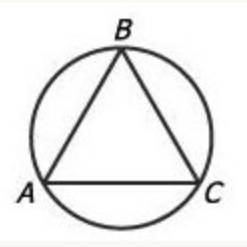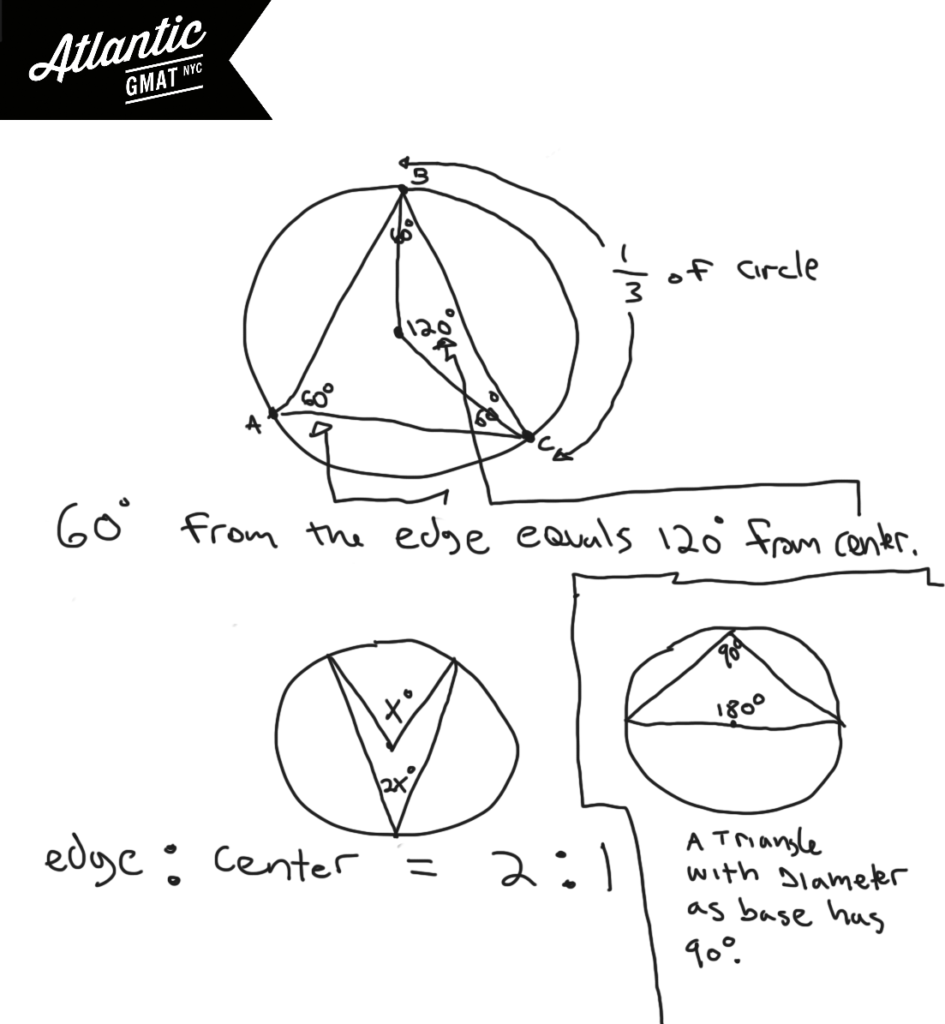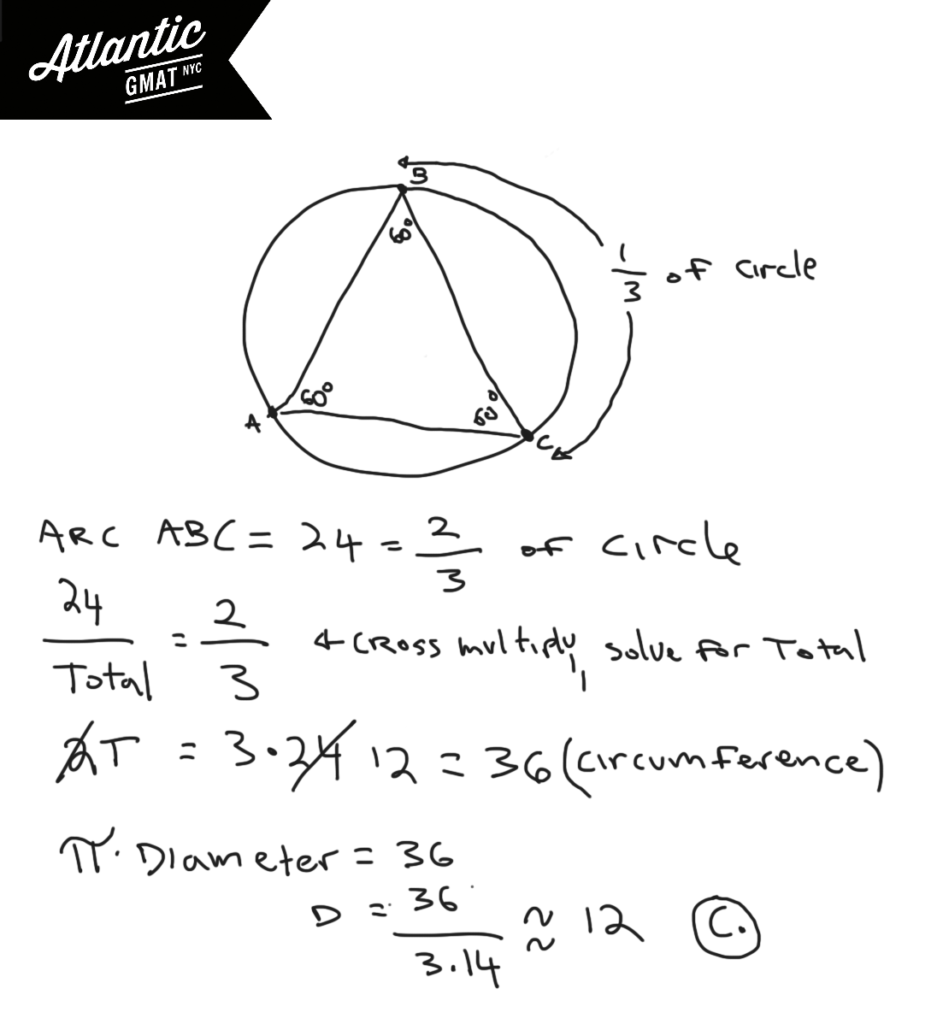Private GMAT Tutoring in NYC & OnlineIn the figure above, equilateral triangle ABC is inscribed in the circle. If the length of arc ABC is 24, what is the approximate diameter of the circle?

(A) 5
(B) 8
(C) 11
(D) 15
(E) 19

It's key to know that an equilateral triangle has equal sides and equal angles measuring 60 degrees each. So each angle of an equilateral triangle inscribed in a circle cuts off 1/3 of the circle. Why is that? Review the basics! An angle on the circumference of a circle cuts off twice that angle's measure. So a 60 degree angle on the edge of a circle cuts off 120 degrees or 1/3 of a circle (120/360 degrees). A 60 degree angle in the center of a circle only cuts off 60 degrees (it doesn't have the extra space to open up). Examples below to illustrate this. Also, there's a special case for triangles that have the diameter of a circle as their base. Because the diameter is a 180 degree central angle, the angle opposite on the circumference of the circle must be 90 degrees. Not relevant for this question but good to know.How much of the circle is arc ABC? It's covering the same part of the circumference as two of the 60 degree angles from triangle ABA. Each of those angles represent 1/3 of the circle so arc ABC is 2/3 of the circle. If 2/3 is 24, 1/3 of the circle's circumference is 12, and the entire circumference is 36. So Pi*diameter = circumference. Pi*D = 36. D = 36/Pi. D = 36/3.14. A little less than 12. 11 is by far the closest answer choice. A little rusty feeling on these GMAT geometry rules? The rules are the easy part of GMAT studying. Get your fundamentals in shape ASAP. Avoid churning through questions before getting your basics GMAT quant organized. You don't want to waste good material and it's much harder to focus on the basics while working on tougher/trickier GMAT questions. Comment with any questions or additions. Happy studies!# CONTACT

Atlantic GMAT

405 East 51st St.

NY, NY 10022

(347) 669-3545

info@AtlanticGMAT.com